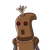# the denominator of fraction is 2 more than numerator. if 1 is subtracted from numerator and 1is added to denominator the fraction

the denominator of fraction is 2 more than numerator. if 1 is subtracted from numerator and 1is added to denominator the fraction becomes 1/2 what is that fraction.​

### 1 thought on “the denominator of fraction is 2 more than numerator. if 1 is subtracted from numerator and 1is added to denominator the fraction”

1.$$\Large {\underline { \sf \orange{Clarification :}}}$$

Here, as per the provided question we are given that the denominator of fraction is 2 more than numerator. Also, if 1 is subtracted from numerator and 1is added to denominator the fraction becomes 1/2. We have to find the out original fraction.

We’ll first assume the numerator and denominator as two variables, say x and y. After that, we’ll form a linear equation and by solving that equation we’ll find the numerator and the denominator.

$$\Large {\underline { \sf \orange{Explication \: of \: Steps :}}}$$

Let,

$$\maltese$$ Numerator = x

$$\maltese$$ Denominator = y

$$\bigstar \: \boxed{\sf { Fraction = \dfrac{x}{y} }} \\$$

According to the question,

$$\longrightarrow$$ Denominator = 2 + Numerator

$$\longrightarrow$$ y = 2 + x

Let it be the equation (1).

Also, as per the question,

• If 1 is subtracted from numerator and 1 is added to denominator the fraction becomes 1/2 what is that fraction.

$$\longrightarrow \sf { \dfrac{x-1}{y+1} = \dfrac{1}{2} }$$

» Substituting the value of y from the equation (1).

$$\longrightarrow \sf { \dfrac{x-1}{2 + x+1} = \dfrac{1}{2} }$$

$$\longrightarrow \sf { \dfrac{x-1}{3 + x} = \dfrac{1}{2} }$$

By using cross multiplication method,

$$\longrightarrow \sf { 2(x-1) = 1(3+x) }$$

Using distributive property,

$$\longrightarrow \sf { 2(x) + 2( -1) = 1(3) +1( x) }$$

Performing multiplication,

$$\longrightarrow \sf { 2x – 2= 3 +x }$$

Transposing variables and constants,

$$\longrightarrow \sf { 2x – x = 3 + 2 }$$

$$\longrightarrow \sf { x = 5 }$$

$$\star \large {\bf{ Numerator = 5}} \star$$

Finding out the denominator :

From the equation (1), we have :

$$\longrightarrow \sf { y = 2 + x }$$

Substituting the value of x,

$$\longrightarrow \sf { y = 2 + 5 }$$

$$\longrightarrow \sf { y = 7 }$$

$$\star \large {\bf{ Denominator = 7}} \star$$

$$\longrightarrow \\$$ ❝ $$\boxed{ \sf \orange { Fraction = \dfrac{5}{7} }} \\$$ ❞

Therefore, the fraction is $$\pmb { \mathfrak \gray { \dfrac{5}{7} }}$$.